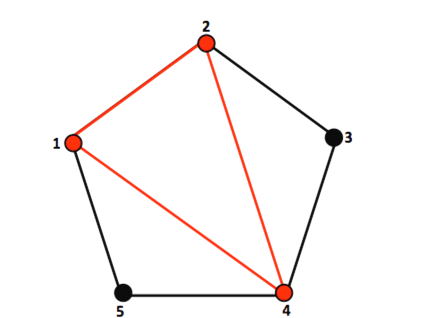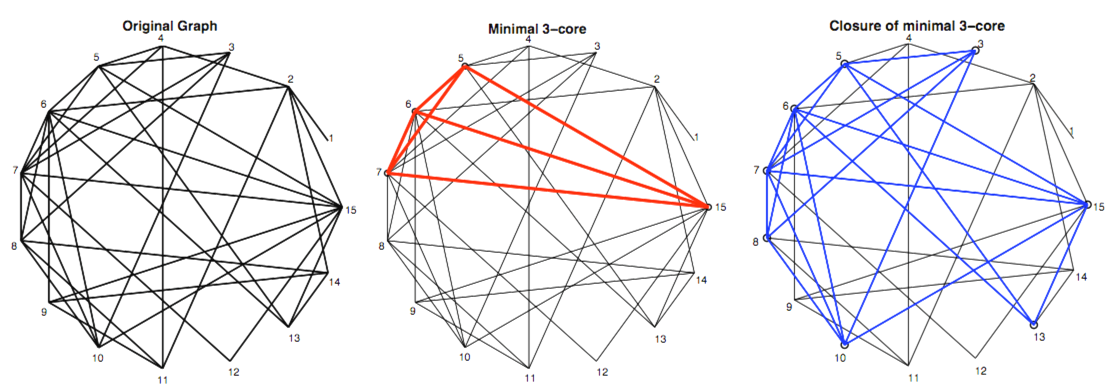# 8.2 Cell assemblies: a binary integer programming problem  (Page 2/2)

 Page 2 / 2

## Threshold ( k )

In undirected, unweighted graphs the threshold( k ) is the minimum number of inputs a neuron needs in order to become excited

## The excitation map, e(x,k)

• let X be a subgraph in G :
${x}_{i}=\left\{\begin{array}{cc}1\hfill & \text{if}\phantom{\rule{4.pt}{0ex}}{v}_{i}\in X\hfill \\ 0\hfill & \text{if}\phantom{\rule{4.pt}{0ex}}\text{otherwise}\hfill \end{array}\right)$
• Where x is a binary vector representing the presence (1) or absence (0) of a neuron.
$e{}_{i}\left(x,k\right)=\left\{\begin{array}{cc}1\hfill & \text{if}\phantom{\rule{4.pt}{0ex}}{\left({A}_{d}x\right)}_{i}\ge k\hfill \\ 0\hfill & \text{if}\phantom{\rule{4.pt}{0ex}}\text{otherwise}\hfill \end{array}\right)$
• x is an invariant set if:
$e\left(x,k\right)=x$

## Graph theory and cell assemblies

Using tools from graph theory, we are able to link a certain class of Palm's cell assemblies, which we will call a k -assembly, to the closure of a minimal k -core. Using MATLAB, we are able to find a minimal k -core and it's closure allowing us to find a k -assembly in any given network of neurons.

## k -cores

k -cores are a concept in graph theory that has been widely used and studied by many graph theorists for locating cohesive subsets in a given graph. A k -core can be defined as:

• Given graph G , subgraph X is a k -core if every node in X has at lease k neighbors in X . A k -core can be described as minimal if no proper subset of the k -core is also a k -core.X = { 1 , 2 , 4 } is a minimal 2-core

## Algorithms for finding a k -assembly

The closure (the invariant set generated by iterating the e map) of a minimal k -core is a k -assembly

• One type of cell assembly, we will call a k -assembly, is the closure of a minimal k -core. In order to find these k -assemblies, we needed to develop a method for finding minimal k -cores and their closures in any given network of neurons.

## Finding minimal k -cores:

bintprog is a built in MATLAB function that Þnds an optimal solution to binary integer programming problems of the following form:

$\underset{x}{min}{f}^{T}x$
$Ax\le b$
$x\in {\left\{0,1\right\}}^{n}$

bintprog arguments: $f,A,b$

• f : coefficients of the variables of the objective functions
• $A,b$ : Using the threshold inequality we can find A :
${A}_{d}x\ge kx⟹0\ge \left(kI-{A}_{d}\right)x$
bintprog minimizes ${f}^{T}x$ constrained to $Ax\le b$ . Because x=0 satisfies the inequality we must add an additional constraint
${x}_{1}+{x}_{2}+...+{x}_{n}\ge 1$
$A=\left(\frac{kI-{A}_{d}}{-1-1-1...}\right)\phantom{\rule{10.pt}{0ex}}$
$b=\left(\begin{array}{c}0\\ 0\\ 0\\ ⋮\\ 0\\ -1\end{array}\right)$

## bintprog Example

• Given the following graph, its corresponding adjacency matrix and threshold of $k=2$ , bintprog can find a minimal 2-core:
${A}_{d}=\left(\begin{array}{cccccc}0& 1& 0& 0& 0& 1\\ 1& 0& 1& 0& 1& 1\\ 0& 1& 0& 1& 1& 1\\ 0& 0& 1& 0& 1& 0\\ 0& 1& 1& 1& 0& 1\\ 1& 1& 1& 0& 1& 0\end{array}\right)$
• bintprog arguments:
$f=\left(\begin{array}{c}1\\ 1\\ 1\\ 1\\ 1\\ 1\end{array}\right)\phantom{\rule{50.pt}{0ex}}A=\left(\begin{array}{cccccc}2& -1& 0& 0& 0& -1\\ -1& 2& -1& 0& -1& -1\\ 0& -1& 2& -1& -1& -1\\ 0& 0& -1& 2& -1& 0\\ 0& -1& -1& -1& 2& -1\\ -1& -1& -1& 0& -1& 2\\ -1& -1& -1& -1& -1& -1\end{array}\right)\phantom{\rule{50.pt}{0ex}}b=\left(\begin{array}{c}0\\ 0\\ 0\\ 0\\ 0\\ 0\\ -1\end{array}\right)$
• bintprog returns a vector, x , indicating which nodes are in the minimal k -core:
$x=\left(\begin{array}{c}1\\ 1\\ 0\\ 0\\ 0\\ 1\end{array}\right)$
• {1,2,6} is the minimal 2-core found by bintprog
• There are, however, many other minimal k -cores in this set of neurons such as: {3,4,5} and {2,5,6}. Our goal for the future is to find some method that enables bintprog to find all of the minimal k -cores in a given network allowing us to find all of the k -assemblies in that network.

## Closure example

We will use the same graph as the previous bintprog example:

1. Create a subset, x . We will use the minimal 2-core found by bintprog :
2. Find ${e}^{1}\left(x,k\right)$ :
$\left(\begin{array}{cccccc}0& 1& 0& 0& 0& 1\\ 1& 0& 1& 0& 1& 1\\ 0& 1& 0& 1& 1& 1\\ 0& 0& 1& 0& 1& 0\\ 0& 1& 1& 1& 0& 1\\ 1& 1& 1& 0& 1& 0\end{array}\right)\left(\begin{array}{c}1\\ 1\\ 0\\ 0\\ 0\\ 1\end{array}\right)=\left(\begin{array}{c}2\\ 2\\ 2\\ 0\\ 2\\ 2\end{array}\right)⟹{e}^{1}\left(x,k\right)=\left(\begin{array}{c}1\\ 1\\ 1\\ 0\\ 1\\ 1\end{array}\right)$
Because ${e}^{1}\ne x$ we must apply ${e}_{i}\left(x,k\right)$ again:
$\left(\begin{array}{cccccc}0& 1& 0& 0& 0& 1\\ 1& 0& 1& 0& 1& 1\\ 0& 1& 0& 1& 1& 1\\ 0& 0& 1& 0& 1& 0\\ 0& 1& 1& 1& 0& 1\\ 1& 1& 1& 0& 1& 0\end{array}\right)\left(\begin{array}{c}1\\ 1\\ 1\\ 0\\ 1\\ 1\end{array}\right)=\left(\begin{array}{c}2\\ 4\\ 3\\ 2\\ 3\\ 4\end{array}\right)⟹{e}^{2}\left(x,k\right)=\left(\begin{array}{c}1\\ 1\\ 1\\ 1\\ 1\\ 1\end{array}\right)$
Even though ${e}^{2}\left(x,k\right)\ne x$ , we can see that because the entire graph is excited, it will keep exciting itself, thus generating an invariant set giving us a k -assembly:
$x=\left(\begin{array}{c}1\\ 1\\ 1\\ 1\\ 1\\ 1\end{array}\right)$

## Finding k -assembliesbintprog found a minimal 3-core { 5 , 6 , 7 , 15 } in the 15 node graph and then finds that minimal 3-core's closure (a k -assembly).

## Future work for finding cell assemblies

In order to find more cell assemblies in any given network of neurons, we have come up with a few methods of finding other minimal k -cores in a network of neurons:

• Alter the arguments of bintprog
• We have altered with the coefficients of the objective function where we would increase the value for nodes already found in a previous minimal k -core. This method has, however, proved to be exhaustive in that we must increase the value of the objective coefficients with all possible combinations of nodes of the previously found minimal k -cores to ensure that all the minimal k -cores of any given graph are located.
• Translate other graph theoretical algorithms to fit minimal k -cores
• We hope to use algorithms in graph theory for finding other types of subgraphs, such as a maximal clique, and translate them to fit our problem of finding all of the minimal k -cores of any given graph.
• Use probability in random graphs
• Using probability may allow us to figure out how many minimal k -cores a given graph may support as well as where they might be, allowing us to constrain other exhaustive methods of finding minimal k -cores.

## Conclusion

This module has shown how to translate the problem of finding cell assemblies in a network of neurons into a binary integer programming problem. It has shown a clear connection between cell assemblies and graph theory and also how to find at least one cell assembly in any given network of neurons. Future work for this problem includes how to find more cell assemblies in a network of neurons.

## Acknowledgements

I would like to give a big thanks to Dr. Steve Cox and Dr. Illya Hicks for guiding us through our project of finding cell assemblies. Also thanks to the REU students, Karina Aliaga, Shaunak Das, and Diane Taylor who I collaborated with on this project. Lastly I would like to thank NSF and the VIGRE program for funding me under the NSF VIGRE Grant DMS-0240058.

1. Hebb, Donald. (1949) The Organization of Behavior. (New York: John Wiley).

2. Palm, Gunther. (1981) Towards a Theory of Cell Assemblies. Biological Cybernetics 39, pp. 181-194.

where we get a research paper on Nano chemistry....?
what are the products of Nano chemistry?
There are lots of products of nano chemistry... Like nano coatings.....carbon fiber.. And lots of others..
learn
Even nanotechnology is pretty much all about chemistry... Its the chemistry on quantum or atomic level
learn
da
no nanotechnology is also a part of physics and maths it requires angle formulas and some pressure regarding concepts
Bhagvanji
Preparation and Applications of Nanomaterial for Drug Delivery
revolt
da
Application of nanotechnology in medicine
what is variations in raman spectra for nanomaterials
I only see partial conversation and what's the question here!
what about nanotechnology for water purification
please someone correct me if I'm wrong but I think one can use nanoparticles, specially silver nanoparticles for water treatment.
Damian
yes that's correct
Professor
I think
Professor
Nasa has use it in the 60's, copper as water purification in the moon travel.
Alexandre
nanocopper obvius
Alexandre
what is the stm
is there industrial application of fullrenes. What is the method to prepare fullrene on large scale.?
Rafiq
industrial application...? mmm I think on the medical side as drug carrier, but you should go deeper on your research, I may be wrong
Damian
How we are making nano material?
what is a peer
What is meant by 'nano scale'?
What is STMs full form?
LITNING
scanning tunneling microscope
Sahil
how nano science is used for hydrophobicity
Santosh
Do u think that Graphene and Fullrene fiber can be used to make Air Plane body structure the lightest and strongest. Rafiq
Rafiq
what is differents between GO and RGO?
Mahi
what is simplest way to understand the applications of nano robots used to detect the cancer affected cell of human body.? How this robot is carried to required site of body cell.? what will be the carrier material and how can be detected that correct delivery of drug is done Rafiq
Rafiq
if virus is killing to make ARTIFICIAL DNA OF GRAPHENE FOR KILLED THE VIRUS .THIS IS OUR ASSUMPTION
Anam
analytical skills graphene is prepared to kill any type viruses .
Anam
Any one who tell me about Preparation and application of Nanomaterial for drug Delivery
Hafiz
what is Nano technology ?
write examples of Nano molecule?
Bob
The nanotechnology is as new science, to scale nanometric
brayan
nanotechnology is the study, desing, synthesis, manipulation and application of materials and functional systems through control of matter at nanoscale
Damian
Is there any normative that regulates the use of silver nanoparticles?
what king of growth are you checking .?
Renato
What fields keep nano created devices from performing or assimulating ? Magnetic fields ? Are do they assimilate ?
why we need to study biomolecules, molecular biology in nanotechnology?
?
Kyle
yes I'm doing my masters in nanotechnology, we are being studying all these domains as well..
why?
what school?
Kyle
biomolecules are e building blocks of every organics and inorganic materials.
Joe
Got questions? Join the online conversation and get instant answers!By Brenna FikeBy OpenStaxBy OpenStaxBy Miranda ReisingBy John GabrieliBy Jonathan LongBy Jugnu KhanBy Richley CrapoBy Maureen MillerBy Janet Forrester